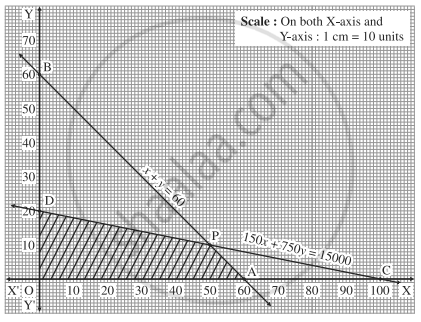# A furniture dealer deals in tables and chairs. He has ₹ 1,50,000 to invest and a space to store at most 60 pieces. A table costs him ₹ 1500 and a chair ₹ 750. - Mathematics and Statistics

Sum

A furniture dealer deals in tables and chairs. He has ₹ 1,50,000 to invest and a space to store at most 60 pieces. A table costs him ₹ 1500 and a chair ₹ 750. Construct the inequations and find the feasible solution.

#### Solution

Let x be the number of tables and y be the number of chairs. Then x ≥ 0, y ≥ 0.

The dealer has a space to store at most 60 pieces. ∴ x + y ≤ 60

Since, the cost of each table is ₹ 1500 and that of each chair is ₹ 750, the total cost of x tables and y chairs is 1500x + 750y. Since the dealer has ₹ 1,50,000 to invest, 1500x + 750y ≤ 1,50,000 = 150x + 750y ≤ 15000 = 2x + y ≤ 200

Hence the system of inequations are

x + y ≤ 60, 2x + y ≤ 200

First we draw the lines AB and CD whose equations are

x + y = 60 and 2x + y ≤ 200, x ≥ 0, y ≥ 0 respectively.

 Line Equation Points on the X-axis Points on the Y-axis Sign Region AB x + y = 60 A(60,0) B(0,60) ≤ origin side of line AB CD 2x + y = 200 C(100,0) D(0,200) ≤ origin side of line CDThe feasible solution is OAPDO which is shaded in the graph.

Concept: Linear Programming Problem (L.P.P.)
Is there an error in this question or solution?
Chapter 7: Linear Programming - Exercise 7.2 [Page 234]

Share